Foxtable(狐表)用户栏目专家坐堂 → 通过临时列积分排名次

共有574人关注过本帖树形打印复制链接

# 主题：通过临时列积分排名次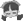1楼 | 信息 | 搜索 | 邮箱 | 主页 | UC加好友发短信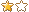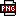此主题相关图片如下：排名.png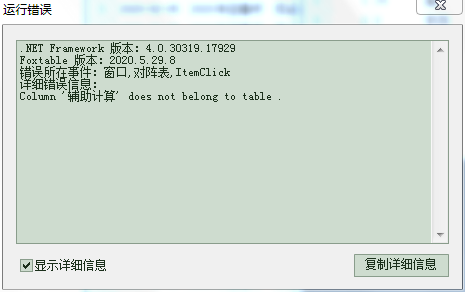下载信息  [文件大小：   下载次数： ]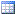点击浏览该文件:象棋排赛（2020706）.foxdb

Dim qsmc As WinForm.ComboBox = e.Form.Controls("棋赛名称")
Dim di1 As String = qsmc.Value
Dim m As WinForm.TextBox = e.Form.Controls("第几轮")
Dim di2 As String = m.Value
Dim bsrq As WinForm.DateTimePicker = e.Form.Controls("rq")
Dim di3 As Date = bsrq.Value
Dim fz As WinForm.ComboBox = e.Form.Controls("fenzhu")
Dim Str As String = fz.Value

If di2 >= 2
If DataTables("比赛积分").DataCols.Contains("辅助计算") = False Then
End If
Dim drs1 As List(of DataRow)  = DataTables("比赛积分").SQLSelect("[棋赛名称] = '" & di1 & "' And [第几轮比赛] = '" & di2 & "' And [分组] = '" & Str & "'","","上轮止累计积分 Desc,曾交战对手累计积分 Desc")
For Each dr1 As DataRow In drs1
dr1("辅助计算") = dr1("上轮止累计积分") + dr1("曾交战对手累计积分")
Next
Dim bjs As List(Of String) = DataTables("比赛积分").SQLGetValues("姓名","[棋赛名称] = '" & di1 & "' And [第几轮比赛] = '" & di2 & "' And [分组] = '" & Str & "'","上轮止累计积分 Desc,曾交战对手累计积分 Desc")
For Each bj As String In bjs
Dim drs2 As List(Of DataRow) = DataTables("比赛积分").Select("[姓名] = " & bj, "辅助计算 DESC")
For n As Integer = 0 To drs2.Count - 1 '遍历所有行
If n > 0 AndAlso drs2(n)("辅助计算") = drs2(n-1)("辅助计算") Then '如果总分和上一行相同
drs2(n)("名次") = drs2(n-1)("名次") '则排名等于上一行
Else
drs2(n)("名次") = n + 1 '设置排名
End If
Next
Next
End If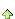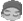2楼 | 信息 | 搜索 | 邮箱 | 主页 | UC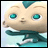加好友发短信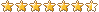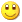Post By：2020/7/6 20:52:00 [只看该作者]

 SQLSelect是从后台数据库取值，后台数据库是不存在临时列的。如果要使用临时列数据只有使用Select3楼 | 信息 | 搜索 | 邮箱 | 主页 | UC加好友发短信Post By：2020/7/6 20:56:00 [只看该作者]

 临时列数据是从后台取数计算出来的，不是直接从后台取，后台没这数据。4楼 | 信息 | 搜索 | 邮箱 | 主页 | UC加好友发短信Post By：2020/7/6 20:58:00 [只看该作者]

 Select不是只能计算已加载数据吗？我是分页加载的，用Select数据不全面吧？5楼 | 信息 | 搜索 | 邮箱 | 主页 | UC加好友发短信Post By：2020/7/6 21:02:00 [只看该作者]

 不要临时列也很简单，在比赛积分表再加一列数值列就行，可我不想要那么多列，想显示简洁点，当然不想看到它隐藏列也可以，现在不是在学编程吗？尽量多学学。6楼 | 信息 | 搜索 | 邮箱 | 主页 | UC加好友发短信Post By：2020/7/6 22:27:00 [只看该作者]

 不要临时列改为在比赛积分表添加一列数值列，Dim qsmc As WinForm.ComboBox = e.Form.Controls("棋赛名称")        Dim di1 As String = qsmc.Value        Dim m As WinForm.TextBox = e.Form.Controls("第几轮")        Dim di2 As String = m.Value        Dim bsrq As WinForm.DateTimePicker = e.Form.Controls("rq")        Dim di3 As Date = bsrq.Value        Dim fz As WinForm.ComboBox = e.Form.Controls("fenzhu")        Dim Str As String = fz.Value        For Each nr As Row In Tables("综合组").Rows            Dim dr As DataRow = DataTables("比赛积分").SQLfind("[棋赛名称] = '" & di1 & "' And [第几轮比赛] = '" & di2 & "' And [分组] = '" & Str & "' And [编号] = '" & nr("红方_编号") & "'")            If dr IsNot Nothing Then                If di3 <> Nothing Then                    dr("日期") = di3                End If                dr("桌号") = nr("桌号")                dr("红黑方") = "红方"                dr("积分") = nr("当前局成绩_红方")                dr.Save            End If            dr = DataTables("比赛积分").SQLfind("[棋赛名称] = '" & di1 & "' And [第几轮比赛] = '" & di2 & "' And [分组] = '" & Str & "' And [编号]='" & nr("黑方_编号") & "'")            If dr IsNot Nothing Then                If di3 <> Nothing Then                    dr("日期") = di3                End If                dr("桌号") = nr("桌号")                dr("红黑方") = "黑方"                dr("积分") = nr("当前局成绩_黑方")                dr.Save            End If            If di2 >= 2                dr("辅助计算") = dr("上轮止累计积分") + dr("积分") + dr("曾交战对手累计积分") + DataTables("比赛积分").SQLCompute("SUM(积分)","[分组] = '" & Str & "' And [桌号] = " & dr("桌号") & " And [编号] <> '" & dr("编号") & "'and [姓名] <> '" & dr("姓名") & "'")            End If        Next        DataTables("比赛积分").load        Dim drs1 As List(of DataRow)  = DataTables("比赛积分").SQLSelect("[棋赛名称] = '" & di1 & "' And [第几轮比赛] = '" & di2 & "' And [分组] = '" & Str & "'","","上轮止累计积分 Desc,曾交战对手累计积分 Desc")        Dim bjs As List(Of String) = DataTables("比赛积分").SQLGetValues("姓名","[棋赛名称] = '" & di1 & "' And [第几轮比赛] = '" & di2 & "' And [分组] = '" & Str & "'","上轮止累计积分 Desc,曾交战对手累计积分 Desc")        For Each bj As String In bjs            Dim drs2 As List(Of DataRow) = DataTables("比赛积分").Select("[姓名] = " & bj, "辅助计算 DESC")            For n As Integer = 0 To drs2.Count - 1 '遍历所有行                If n > 0 AndAlso drs2(n)("辅助计算") = drs2(n-1)("辅助计算") Then '如果总分和上一行相同                    drs2(n)("名次") = drs2(n-1)("名次") '则排名等于上一行                Else                    drs2(n)("名次") = n + 1 '设置排名                End If            Next        NextEnd Select此主题相关图片如下：qq图片20200706222009.png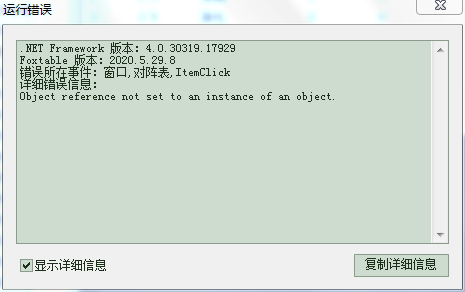辅助计算列并没有数值。7楼 | 信息 | 搜索 | 邮箱 | 主页 | UC加好友发短信Post By：2020/7/6 22:41:00 [只看该作者]

 If dr IsNot Nothing Then                If di3 <> Nothing Then                    dr("日期") = di3                End If                dr("桌号") = nr("桌号")                dr("红黑方") = "黑方"                dr("积分") = nr("当前局成绩_黑方")            If di2 >= 2                dr("辅助计算") = dr("上轮止累计积分") + dr("积分") + dr("曾交战对手累计积分") + DataTables("比赛积分").SQLCompute("SUM(积分)","[分组] = '" & Str & "' And [桌号] = " & dr("桌号") & " And [编号] <> '" & dr("编号") & "'and [姓名] <> '" & dr("姓名") & "'")            End If                dr.Save            End If8楼 | 信息 | 搜索 | 邮箱 | 主页 | UC加好友发短信Post By：2020/7/7 10:01:00 [只看该作者]

 辅助计算列的数值没问题了，用注识的代码，报错，用没注识代码生成的名次不对：此主题相关图片如下：2112.png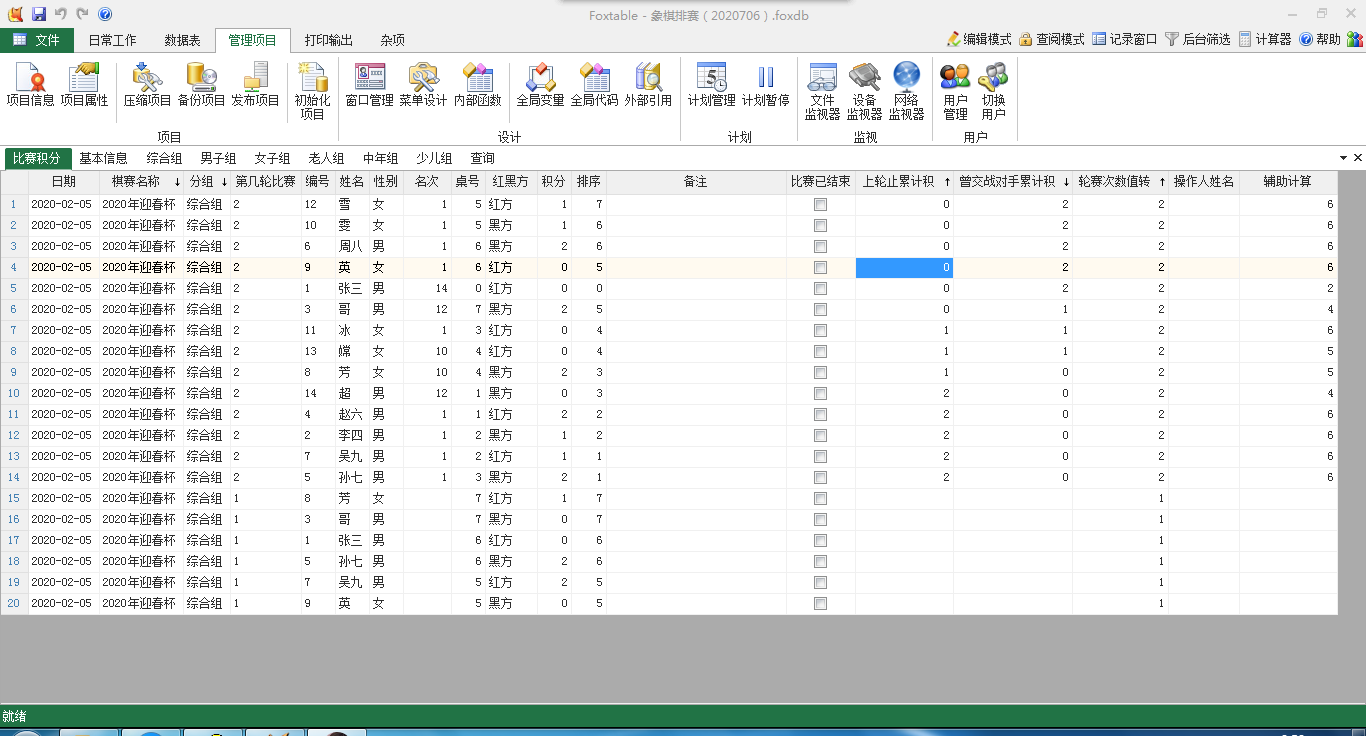Dim qsmc As WinForm.ComboBox = e.Form.Controls("棋赛名称")        Dim di1 As String = qsmc.Value        Dim m As WinForm.TextBox = e.Form.Controls("第几轮")        Dim di2 As String = m.Value        Dim bsrq As WinForm.DateTimePicker = e.Form.Controls("rq")        Dim di3 As Date = bsrq.Value        Dim fz As WinForm.ComboBox = e.Form.Controls("fenzhu")        Dim Str As String = fz.Value        For Each nr As Row In Tables("综合组").Rows            Dim dr As DataRow = DataTables("比赛积分").SQLfind("[棋赛名称] = '" & di1 & "' And [第几轮比赛] = '" & di2 & "' And [分组] = '" & Str & "' And [编号] = '" & nr("红方_编号") & "'")            If dr IsNot Nothing Then                If di3 <> Nothing Then                    dr("日期") = di3                End If                dr("桌号") = nr("桌号")                dr("红黑方") = "红方"                dr("积分") = nr("当前局成绩_红方")                If di2 >= 2                    dr("辅助计算") = dr("上轮止累计积分") + dr("积分") + dr("曾交战对手累计积分") + DataTables("比赛积分").SQLCompute("SUM(积分)","[分组] = '" & Str & "' And [桌号] = " & dr("桌号") & " And [编号] <> '" & dr("编号") & "'and [姓名] <> '" & dr("姓名") & "'")                End If                dr.Save            End If            dr = DataTables("比赛积分").SQLfind("[棋赛名称] = '" & di1 & "' And [第几轮比赛] = '" & di2 & "' And [分组] = '" & Str & "' And [编号]='" & nr("黑方_编号") & "'")            If dr IsNot Nothing Then                If di3 <> Nothing Then                    dr("日期") = di3                End If                dr("桌号") = nr("桌号")                dr("红黑方") = "黑方"                dr("积分") = nr("当前局成绩_黑方")                If di2 >= 2                    dr("辅助计算") = dr("上轮止累计积分") + dr("积分") + dr("曾交战对手累计积分") + DataTables("比赛积分").SQLCompute("SUM(积分)","[分组] = '" & Str & "' And [桌号] = " & dr("桌号") & " And [编号] <> '" & dr("编号") & "'and [姓名] <> '" & dr("姓名") & "'")                End If                dr.Save            End If        Next        DataTables("比赛积分").load'Dim bjs As List(Of String) = DataTables("比赛积分").SQLGetValues("姓名","[棋赛名称] = '" & di1 & "'And [第几轮比赛] = '" & di2 & "' And [分组] = '" & Str & "'")'For Each bj As String In bjs'Dim drs As List(Of DataRow) = DataTables("比赛积分").Select("[姓名] = " & bj, "辅助计算 DESC")    'For n As Integer = 0 To drs.Count - 1 '遍历所有行        'If n > 0 AndAlso drs(n)("辅助计算") = drs(n-1)("辅助计算") Then '如果总分和上一行相同            'drs(n)("名次") = drs(n-1)("名次") '则排名等于上一行        'Else            'drs(n)("名次") = n + 1 '设置排名        'End If    'Next        Dim drs2 As List(Of String) = DataTables("比赛积分").SQLSelect("[棋赛名称] = '" & di1 & "'And [第几轮比赛] = '" & di2 & "' And [分组] = '" & Str & "'")        Dim drs1 As List(of DataRow) = DataTables("比赛积分").SQLSelect("[棋赛名称] = '" & di1 & "'And [第几轮比赛] = '" & di2 & "' And [分组] = '" & Str & "'","","辅助计算 DESC")        For n As Integer = 0 To drs1.Count - 1 '遍历所有行            If n > 0 AndAlso drs1(n)("辅助计算") = drs1(n-1)("辅助计算") Then '如果总分和上一行相同                drs2(n)("名次") = drs1(n-1)("名次") '则排名等于上一行            Else                drs2(n)("名次") = n + 1 '设置排名            End If        Next        DataTables("比赛积分").load9楼 | 信息 | 搜索 | 邮箱 | 主页 | UC加好友发短信Post By：2020/7/7 10:10:00 [只看该作者]

 Dim drs As List(Of DataRow) = DataTables("比赛积分").Select("[姓名] = '" & bj & "'", "辅助计算 DESC")10楼 | 信息 | 搜索 | 邮箱 | 主页 | UC加好友发短信Post By：2020/7/7 10:31:00 [只看该作者]

 改为这样名次全为1                Dim qsmc As WinForm.ComboBox = e.Form.Controls("棋赛名称")        Dim di1 As String = qsmc.Value        Dim m As WinForm.TextBox = e.Form.Controls("第几轮")        Dim di2 As String = m.Value        Dim bsrq As WinForm.DateTimePicker = e.Form.Controls("rq")        Dim di3 As Date = bsrq.Value        Dim fz As WinForm.ComboBox = e.Form.Controls("fenzhu")        Dim Str As String = fz.Value        For Each nr As Row In Tables("综合组").Rows            Dim dr As DataRow = DataTables("比赛积分").SQLfind("[棋赛名称] = '" & di1 & "' And [第几轮比赛] = '" & di2 & "' And [分组] = '" & Str & "' And [编号] = '" & nr("红方_编号") & "'")            If dr IsNot Nothing Then                If di3 <> Nothing Then                    dr("日期") = di3                End If                dr("桌号") = nr("桌号")                dr("红黑方") = "红方"                dr("积分") = nr("当前局成绩_红方")                If di2 >= 2                    dr("辅助计算") = dr("上轮止累计积分") + dr("积分") + dr("曾交战对手累计积分") + DataTables("比赛积分").SQLCompute("SUM(积分)","[分组] = '" & Str & "' And [桌号] = " & dr("桌号") & " And [编号] <> '" & dr("编号") & "'and [姓名] <> '" & dr("姓名") & "'")                End If                dr.Save            End If            dr = DataTables("比赛积分").SQLfind("[棋赛名称] = '" & di1 & "' And [第几轮比赛] = '" & di2 & "' And [分组] = '" & Str & "' And [编号]='" & nr("黑方_编号") & "'")            If dr IsNot Nothing Then                If di3 <> Nothing Then                    dr("日期") = di3                End If                dr("桌号") = nr("桌号")                dr("红黑方") = "黑方"                dr("积分") = nr("当前局成绩_黑方")                If di2 >= 2                    dr("辅助计算") = dr("上轮止累计积分") + dr("积分") + dr("曾交战对手累计积分") + DataTables("比赛积分").SQLCompute("SUM(积分)","[分组] = '" & Str & "' And [桌号] = " & dr("桌号") & " And [编号] <> '" & dr("编号") & "'and [姓名] <> '" & dr("姓名") & "'")                End If                dr.Save            End If        Next        DataTables("比赛积分").loadDim bjs As List(Of String) = DataTables("比赛积分").SQLGetValues("姓名","[棋赛名称] = '" & di1 & "'And [第几轮比赛] = '" & di2 & "' And [分组] = '" & Str & "'")For Each bj As String In bjsDim drs As List(Of DataRow) = DataTables("比赛积分").Select("[姓名] = '" & bj & "'", "辅助计算 DESC")    For n As Integer = 0 To drs.Count - 1 '遍历所有行        If n > 0 AndAlso drs(n)("辅助计算") = drs(n-1)("辅助计算") Then '如果总分和上一行相同            drs(n)("名次") = drs(n-1)("名次") '则排名等于上一行        Else            drs(n)("名次") = n + 1 '设置排名        End If    NextNext总数 31 1 2 3 4 下一页